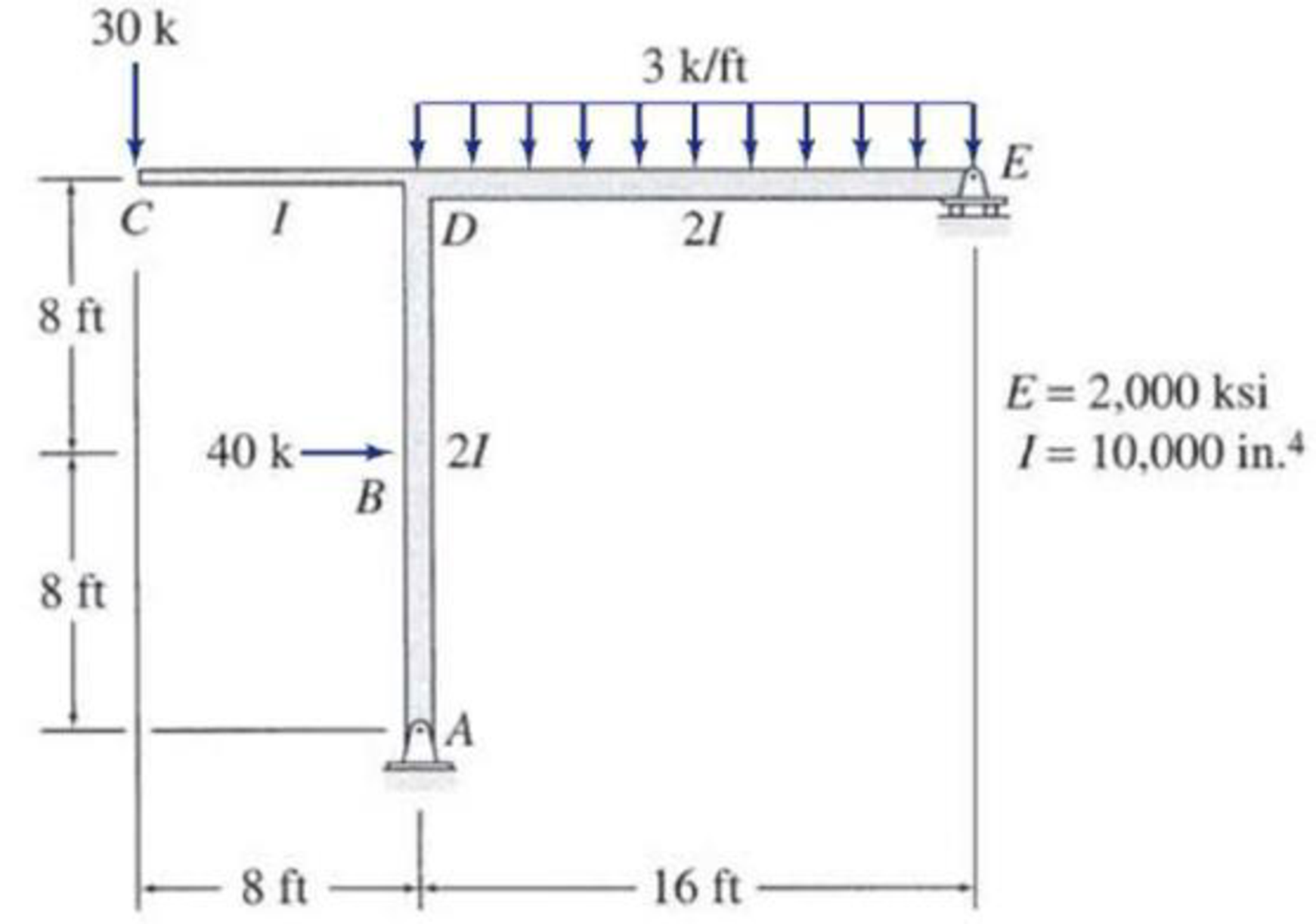# Use the virtual work method to determine the horizontal deflection at joint E of the frame shown in Fig. P7.39. FIG. P7.39, P7.40

#### Solutions

Chapter
Section
Chapter 7, Problem 40P
Textbook Problem
54 views

## Use the virtual work method to determine the horizontal deflection at joint E of the frame shown in Fig. P7.39.FIG. P7.39, P7.40

To determine

Find the horizontal deflection at joint E of the frame using virtual work method.

### Explanation of Solution

Given information:

The frame is given in the Figure.

The value of E is 2,000 ksi and I is 10,000in.4

Apply the sign conventions for calculating reactions, forces and moments using the three equations of equilibrium as shown below.

• For summation of forces along x-direction is equal to zero (Fx=0), consider the forces acting towards right side as positive (+) and the forces acting towards left side as negative ().
• For summation of forces along y-direction is equal to zero (Fy=0), consider the upward force as positive (+) and the downward force as negative ().
• For summation of moment about a joint is equal to zero (Matapoint=0), consider the clockwise moment as negative and the counter clockwise moment as positive.

Calculation:

Consider the real system.

Draw a diagram showing all the given real loads acting on it.

Let the bending moment due to real load be M.

Sketch the real system of the frame as shown in Figure 1.

Summation of moments about A is equal to 0.

MA=0Ey(16)3(16)(162)+30(8)40(8)=0Ey=29k

Summation of forces along y-direction is equal to 0.

+Fy=0Ay+Ey3(16)30=0Ay+293(16)30=0Ay=49k

Summation of forces along x-direction is equal to 0.

+Fx=0Ax+40=0Ax=40k

Consider the virtual system.

Draw a diagram of frame without the given real loads. For horizontal deflection apply unit load at the joint in the horizontal direction.

Let the bending moment due to virtual load be Mv.

Sketch the virtual system of the frame with unit load at joint E as shown in Figure 2.

Find the reactions at the supports A and E:

Summation of moments about A is equal to 0.

MA=0Ey(16)1(16)=0Ey=1k

Summation of forces along y-direction is equal to 0.

+Fy=0Ay+Ey=0Ay+1=0Ay=1k

Summation of forces along x-direction is equal to 0.

+Fy=0Ax+1=0Ax=1k

Find the equations for M and Mv for the 3 segments of the frame as shown in Table 1.

 Segment x-coordinate M (k-ft) Mv (k-ft) Origin Limits (ft) AB A 0−8 40x 1x BD A 8−16 40x−40(x−8)=320 1x ED E 0−16 29x−3x22 1x

Find the horizontal deflection at E using the virtual work expression:

1(ΔE)=0LMvMEIdx (1)

For span AB, BD, and ED the moment of inertia is 2I

### Still sussing out bartleby?

Check out a sample textbook solution.

See a sample solution

#### The Solution to Your Study Problems

Bartleby provides explanations to thousands of textbook problems written by our experts, many with advanced degrees!

Get Started

Find more solutions based on key concepts
For Problems 16.33 through 16.38, draw the isometric view of the following objects. Make the necessary measurem...

Engineering Fundamentals: An Introduction to Engineering (MindTap Course List)

What formula can a project manager use to estimate task duration? Provide an example.

Systems Analysis and Design (Shelly Cashman Series) (MindTap Course List)

How would you define artificial intelligence?

Principles of Information Systems (MindTap Course List)

Are passphrases more secure than passwords? Why or why not? (223)

Enhanced Discovering Computers 2017 (Shelly Cashman Series) (MindTap Course List)

Why is the torch tip raised as the cutting lever is depressed when cutting a hole?

Welding: Principles and Applications (MindTap Course List)

If your motherboard supports ECC DDR3 memory, can you substitute non-ECC DDR3 memory?

A+ Guide to Hardware (Standalone Book) (MindTap Course List)